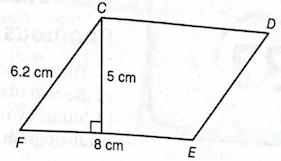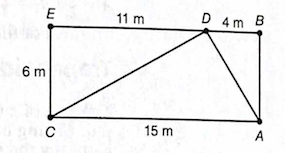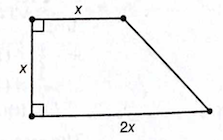## Labels

### GED Math Review: Perimeter and Area of Triangles and Quadrilateral

Questions:

1. A rectangular room is 4.5 meters by 12 meters. What is the area of the room in square meters?
• 27 m2
• 33 m2
• 48 m2
• 54 m2
2. The area of a square is 36 square inches. What is the length of a side of the square?
• 6 in.
• 9 in.
• 18 in.
• Cannot be determined from the given information.
3. The city wants to preserve a rectangular mural that is 32 feet by 15 feet. One quart of protective covers 100 square feet. How many quarts should the city buy to cover the mural?
• 4
• 5
• 6
• 7
4. The base and height of a rectangle are both doubled. What happens to the area of the rectangle?
• It remains the same.
• It is multiplied by 2.
• It is multiplied by 4.
• This cannot be determined from the given information.
5. A contractor is tiling a kitchen floor that measures 12 feet by 18 feet. If each tile measures 2 feet by 3 feet, how many tiles are needed to cover the floor?
Find the area of parallelogram CDEF.• 28.4 cm2
• 31 cm2
• 40 cm2
• 49.6 cm2
6. If figure ABEC is a rectangle, find the area of triangle ACD.• 27 m2
• 45 m2
• 66 m2
• 90 m2
7. Complete the equation that expresses the area of the figure below in terms of x.The area is A = __________x2.

1. DOK Level 1; Content Topic: Q.4.a
The area of the rectangle is

A = bh

A = (4.5m)(12 m)
A = 54m2

2. DOK Level 1; Content Topic: Q.4.a
The area of the square is
A = s2

A = 36 in2, and then s = 6 in

3. DOK Level 2; Content Topic: Q.4.a
The area of the mural is

A = bh

A = (15 ft) (32 ft)
A = 480 ft2
Each quart covers 100 ft2, so it will take 5 quarts of coating to complete the job.

4. DOK Level 2; Content Topic: Q.4.a
The area of a rectangle is

A = bh.

If the base and height are doubled, the area becomes
A = (2b)(2h)
A = 4bh.
This is 4 times the original area.

5. DOK Level 2; Content Topic: Q.4.a
36
The area of the floor is 216 square feet, and the area of each tile is 6 square feet. Since each dimension of a tile is a factor of the corresponding dimension of the floor and 216 divided by 6 is equal to 36, it will take 36 tiles to cover the floor.

6. DOK Level 2; Content Topic: Q.4.a
The area of the parallelogram is

A = bh

A = (8 cm)(5 cm)
A = 40 cm2

7. DOK Level 2; Content Topic: Q.4.a
The area of the triangle is

A = ½ bh

A = ½ (15 m)(6 m)
A = 45 m2

8. 3/2 or 1.5 (DOK Level 2; Content Topic: Q.4.a)
Divide the figure into a square and a right triangle. The area of the square is A = x2and the area of the triangle is

A = ½ bh

A = ½ (x)(x)
A = ½ x2
The total area is A = 3/2 x2.

Reference:

Complete Test Preparation for the GED Test 2014 by Steck-Vaughn